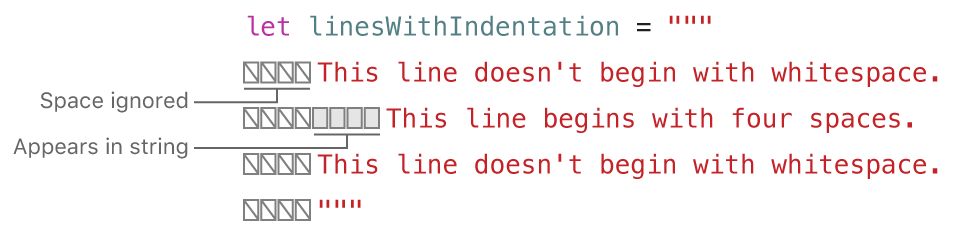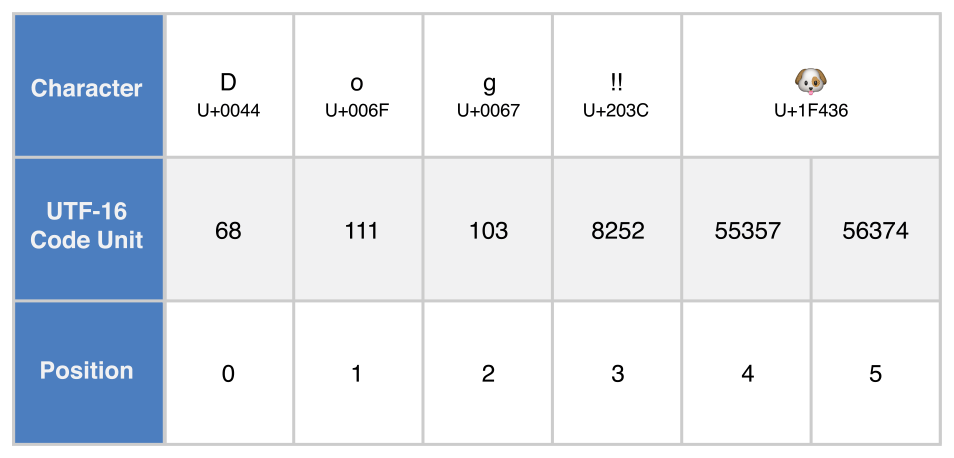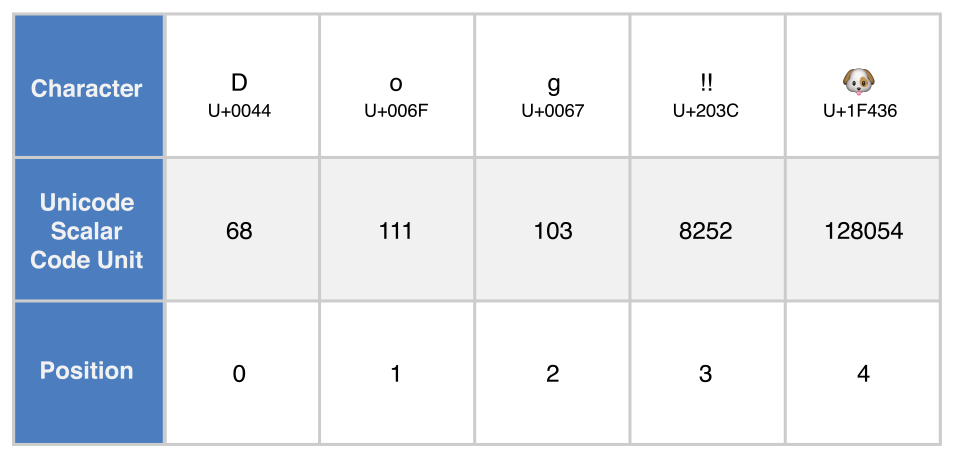# Swift学习之路-字符串和字符

Swift 的 `String``Character` 类型提供了一种快速的符合 Unicode 的方式操作你的代码。字符串的创建和修改语法非常轻量易读，使用与 C 类似的字符串字面量语法。字符串串联只需要使用 `+` 运算符即可，字符串的可修改能力通过选择常量和变量来进行管理，就如同 Swift 语言中的其他值。你同样可以使用字符串来插入常量、变量、字面量以及表达式到更长的字符串当中，这就是所谓的字符串插值。这样让创建自定义字符串值来显示、储存和打印值变得更加简单。

Swift 的`String`类型桥接到了基础库中的`NSString`类。Foundation 同时也扩展了所有 `NSString` 定义的方法给 `String` 。也就是说，如果你导入 Foundation ，就可以在 `String` 中访问所有的 `NSString` 方法，无需转换格式。

## 字符串字面量

``let someString = "Some string literal value"``

### 多行字符串字面量

``````let quotation = """
The White Rabbit put on his spectacles.  "Where shall I begin,

"Begin at the beginning," the King said gravely, "and go on
till you come to the end; then stop."
"""``````

``````let singleLineString = "These are the same."
let multilineString = """
These are the same.
"""``````

``````let softWrappedQuotation = """
The White Rabbit put on his spectacles.  "Where shall I begin, \

"Begin at the beginning," the King said gravely, "and go on \
till you come to the end; then stop."
"""``````

``````let lineBreaks = """

This string starts with a line break.
It also ends with a line break.

"""``````

``````func generateQuotation() -> String {
let quotation = """
The White Rabbit put on his spectacles.  "Where shall I begin,

"Begin at the beginning," the King said gravely, "and go on
till you come to the end; then stop."
"""
return quotation
}
print(quotation == generateQuotation())
// Prints "true"``````### 字符串字面量里的特殊字符

• 转义特殊字符 `\0` (空字符)， `\\` (反斜杠)， `\t` (水平制表符)， `\n` (换行符)， `\r`(回车符)， `\"` (双引号) 以及 `\'` (单引号)；
• 任意的 Unicode 标量，写作 `\u{n}`，里边的 `n`是一个 1-8 个与合法 Unicode 码位相等的16进制数字。

``````let wiseWords = "\"Imagination is more important than knowledge\" - Einstein"
// "Imagination is more important than knowledge" - Einstein
let dollarSign = "\u{24}" // \$, Unicode scalar U+0024
let blackHeart = "\u{2665}" // ♥, Unicode scalar U+2665
let sparklingHeart = "\u{1F496}" // 💖️, Unicode scalar U+1F496``````

``````let threeDoubleQuotationMarks = """
Escaping the first quotation mark \"""
Escaping all three quotation marks \"\"\"
"""``````

### 扩展字符串分隔符

``````let threeMoreDoubleQuotationMarks = #"""
Here are three more double quotes: """
"""#``````

## 初始化一个空字符串

``````var emptyString = ""               // empty string literal
var anotherEmptyString = String()  // initializer syntax
// these two strings are both empty, and are equivalent to each other``````

``````if emptyString.isEmpty {
print("Nothing to see here")
}
// prints "Nothing to see here"``````

## 字符串可变性

``````var variableString = "Horse"
variableString += " and carriage"
// variableString is now "Horse and carriage"

let constantString = "Highlander"
constantString += " and another Highlander"
// this reports a compile-time error - a constant string cannot be modified``````

## 字符串是值类型

Swift 的 `String`类型是一种值类型。如果你创建了一个新的 `String`值， `String`值在传递给方法或者函数的时候会被复制过去，还有赋值给常量或者变量的时候也是一样。每一次赋值和传递，现存的 `String`值都会被复制一次，传递走的是拷贝而不是原本。值类型在结构体和枚举是值类型一章当中有详细描述。

Swift 的默认拷贝 `String`行为保证了当一个方法或者函数传给你一个 `String`值，你就绝对拥有了这个 `String`值，无需关心它从哪里来。你可以确定你传走的这个字符串除了你自己就不会有别人改变它。

## 操作字符

``````for character in "Dog!🐶️" {
print(character)
}
// D
// o
// g
// !
// 🐶️``````

``let exclamationMark: Character = "!"``

`String`值可以通过传入 `Character`值的字符串作为实际参数到它的初始化器来构造：

``````let catCharacters: [Character] = ["C", "a", "t", "!", "🐱️"]
let catString = String(catCharacters)
print(catString)
// prints "Cat!🐱️"``````

## 连接字符串和字符

`String`值能够被加起来（或者说连接），使用加运算符（ +）来创建新的`String`值：

``````let string1 = "hello"
let string2 = " there"
var welcome = string1 + string2
// welcome now equals "hello there"``````

``````var instruction = "look over"
instruction += string2
// instruction now equals "look over there"``````

``````let exclamationMark: Character = "!"
welcome.append(exclamationMark)
// welcome now equals "hello there!"``````

``````let badStart = """
one
two
"""
let end = """
three
"""
// Prints two lines:
// one
// twothree

let goodStart = """
one
two

"""
print(goodStart + end)
// Prints three lines:
// one
// two
// three``````

## 字符串插值

``````let multiplier = 3
let message = "\(multiplier) times 2.5 is \(Double(multiplier) * 2.5)"
// message is "3 times 2.5 is 7.5"``````

`multiplier`的值同时也是字符串后边更大一点表达式的一部分。这个表达式计算了 `Double(multiplier) * 2.5`的值并且插入结果 ( `7.5` ) 到字符串当中。在这个栗子当中，表达式在字符串字面量中被写作 `\(Double(multiplier) * 2.5)` 的形式。

``````print(#"Write an interpolated string in Swift using \(multiplier)."#)
// Prints "Write an interpolated string in Swift using \(multiplier)."``````

``````print(#"6 times 7 is \#(6 * 7)."#)
// Prints "6 times 7 is 42."``````

## Unicode

Unicode 是一种在不同书写系统中编码、表示和处理文本的国际标准。它允许你表示几乎标准化格式的任何语言中的任何字符，并且为外部源比如文本文档或者网页读写这些字符。如同这节中描述的那样，Swift 的 `String``Character`类型是完全 Unicode 兼容的。

### Unicode 标量

Unicode 标量码位位于 `U+0000``U+D7FF`或者 `U+E000``U+10FFFF`之间。Unicode 标量码位不包括从 `U+D800``U+DFFF`的16位码元码位。

### 扩展字形集群

``````let eAcute: Character = "\u{E9}" // é
let combinedEAcute: Character = "\u{65}\u{301}" // e followed by ́
// eAcute is é, combinedEAcute is é``````

``````let precomposed: Character = "\u{D55C}" // 한
let decomposed: Character = "\u{1112}\u{1161}\u{11AB}" // ᄒ, ᅡ, ᆫ
// precomposed is 한, decomposed is 한``````

``````let enclosedEAcute: Character = "\u{E9}\u{20DD}"
// enclosedEAcute is é⃝``````

``````let regionalIndicatorForUS: Character = "\u{1F1FA}\u{1F1F8}"
// regionalIndicatorForUS is 🇺🇸``````

## 字符统计

``````let unusualMenagerie = "Koala 🐨, Snail 🐌, Penguin 🐧, Dromedary 🐪"
print("unusualMenagerie has \(unusualMenagerie.count) characters")
// Prints "unusualMenagerie has 40 characters"``````

``````var word = "cafe"
print("the number of characters in \(word) is \(word.count)")
// Prints "the number of characters in cafe is 4"

word += "\u{301}"    // COMBINING ACUTE ACCENT, U+0301

print("the number of characters in \(word) is \(word.count)")
// Prints "the number of characters in café is 4"``````

## 访问和修改字符串

### 字符串索引

``````let greeting = "Guten Tag!"
greeting[greeting.startIndex]
// G
greeting[greeting.index(before: greeting.endIndex)]
// !
greeting[greeting.index(after: greeting.startIndex)]
// u
let index = greeting.index(greeting.startIndex, offsetBy: 7)
greeting[index]
// a``````

``````greeting[greeting.endIndex] // error
greeting.index(after: endIndex) // error``````

``````for index in greeting.indices {
print("\(greeting[index]) ", terminator: "")
}
// Prints "G u t e n   T a g ! "``````

### 插入和删除

``````var welcome = "hello"
welcome.insert("!", at: welcome.endIndex)
// welcome now equals "hello!"

welcome.insert(contentsOf: " there", at: welcome.index(before: welcome.endIndex))
// welcome now equals "hello there!"``````

``````welcome.remove(at: welcome.index(before: welcome.endIndex))
// welcome now equals "hello there"

let range = welcome.index(welcome.endIndex, offsetBy: -6)..<welcome.endIndex
welcome.removeSubrange(range)
// welcome now equals "hello"``````

## 子字符串

``````let greeting = "Hello, world!"
let index = greeting.index(of: ",") ?? greeting.endIndex
let beginning = greeting[..<index]
// beginning is "Hello"

// Convert the result to a String for long-term storage.
let newString = String(beginning)```````String``Substring` 都遵循 StringProtocol 协议，也就是说它基本上能很方便地兼容所有接受 `StringProtocol` 值的字符串操作函数。你可以无差别使用 `String``Substring` 值来调用这些函数。

## 字符串比较

Swift 提供了三种方法来比较文本值：字符串和字符相等性，前缀相等性以及后缀相等性。

### 字符串和字符相等性

``````let quotation = "We're a lot alike, you and I."
let sameQuotation = "We're a lot alike, you and I."
if quotation == sameQuotation {
print("These two strings are considered equal")
}
// prints "These two strings are considered equal"``````

``````// "Voulez-vous un café?" using LATIN SMALL LETTER E WITH ACUTE
let eAcuteQuestion = "Voulez-vous un caf\u{E9}?"

// "Voulez-vous un café?" using LATIN SMALL LETTER E and COMBINING ACUTE ACCENT
let combinedEAcuteQuestion = "Voulez-vous un caf\u{65}\u{301}?"

if eAcuteQuestion == combinedEAcuteQuestion {
print("These two strings are considered equal")
}
// prints "These two strings are considered equal"``````

``````let latinCapitalLetterA: Character = "\u{41}"

let cyrillicCapitalLetterA: Character = "\u{0410}"

if latinCapitalLetterA != cyrillicCapitalLetterA {
print("These two characters are not equivalent")
}
// prints "These two characters are not equivalent"``````

### 前缀和后缀相等性

``````let romeoAndJuliet = [
"Act 1 Scene 1: Verona, A public place",
"Act 1 Scene 2: Capulet's mansion",
"Act 1 Scene 3: A room in Capulet's mansion",
"Act 1 Scene 4: A street outside Capulet's mansion",
"Act 1 Scene 5: The Great Hall in Capulet's mansion",
"Act 2 Scene 1: Outside Capulet's mansion",
"Act 2 Scene 2: Capulet's orchard",
"Act 2 Scene 3: Outside Friar Lawrence's cell",
"Act 2 Scene 4: A street in Verona",
"Act 2 Scene 5: Capulet's mansion",
"Act 2 Scene 6: Friar Lawrence's cell"
]``````

``````var act1SceneCount = 0
for scene in romeoAndJuliet {
if scene.hasPrefix("Act 1 ") {
act1SceneCount += 1
}
}
print("There are \(act1SceneCount) scenes in Act 1")
// Prints "There are 5 scenes in Act 1"``````

``````var mansionCount = 0
var cellCount = 0
for scene in romeoAndJuliet {
if scene.hasSuffix("Capulet's mansion") {
mansionCount += 1
} else if scene.hasSuffix("Friar Lawrence's cell") {
cellCount += 1
}
}
print("\(mansionCount) mansion scenes; \(cellCount) cell scenes")
// Prints "6 mansion scenes; 2 cell scenes"``````

## 字符串的 Unicode 表示法

Swift 提供了几种不同的方法来访问字符串的 Unicode 表示。你可以使用 `for-in`语句来遍历整个字符串，来访以 Unicode 扩展字形集群的方式访问单独的 `Character`值。这个过程在操作字符章节有着详细的描述。

• UTF-8 码元的集合（关联于字符串的 utf8  属性）
• UTF-16 码元的集合（关联于字符串的 utf16  属性）
• 21位 Unicode 标量值的集合，等同于字符串的 UTF-32 编码格式（关联于字符串的 `unicodeScalars` 属性）

``let dogString = "Dog‼🐶"``

### UTF-8 表示法``````for codeUnit in dogString.utf8 {
print("\(codeUnit) ", terminator: "")
}
print("")
// 68 111 103 226 128 188 240 159 144 182``````

### UTF-16 表示法``````for codeUnit in dogString.utf16 {
print("\(codeUnit) ", terminator: "")
}
print("")
// Prints "68 111 103 8252 55357 56374 "``````

### Unicode 标量表示法``````for scalar in dogString.unicodeScalars {
print("\(scalar.value) ", terminator: "")
}
print("")
// Prints "68 111 103 8252 128054 "``````

``````for scalar in dogString.unicodeScalars {
print("\(scalar) ")
}
// D
// o
// g
// ‼
// 🐶``````